Next: Antenna Directivity and Effective Up: Radiation and Scattering Previous: Introduction

# Basic Antenna Theory

It possible to solve exactly for the radiation pattern emitted by a linear antenna fed with a sinusoidal current pattern. Assuming that all fields and currents vary in time like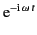, and adopting the Lorenz gauge, it is easily demonstrated that the vector potential obeys the inhomogeneous Helmholtz equation,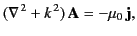(1213)

where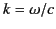. The Green's function for this equation, subject to the Sommerfeld radiation condition (which ensures that sources radiate waves instead of absorbing them), is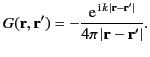(1214)

(See Chapter 1.) Thus, we can invert Equation (1215) to obtain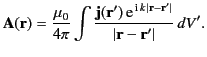(1215)

The electric field in the source-free region,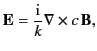(1216)

follows from the Ampère-Maxwell equation, as well as the definition,

Now,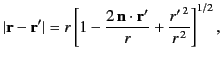(1217)

where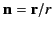. Assuming that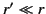, this expression can be expanded binomially to give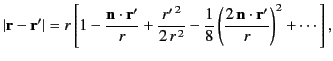(1218)

where we have retained all terms up to order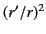. The above expansion, which appears in the complex exponential of Equation (1217), determines the phase of the radiation emitted by each element of the antenna. The quadratic terms in the expansion can be neglected provided they can be shown to contribute a phase change that is significantly less than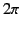. Thus, because the maximum possible value of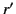is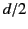, for a linear antenna that extends along the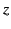-axis from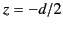to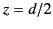, the phase shift associated with the quadratic terms is insignificant as long as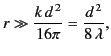(1219)

where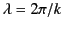is the wavelength of the radiation. This constraint is known as the Fraunhofer limit.

In the Fraunhofer limit, we can approximate the phase variation of the complex exponential in Equation (1217) as a linear function of: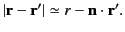(1220)

The denominator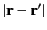in the integrand of Equation (1217) can be approximated as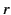provided that the distance from the antenna is much greater than its length: that is,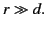(1221)

Thus, Equation (1217) reduces to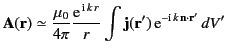(1222)

when the constraints (1221) and (1223) are satisfied. If the additional constraint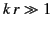(1223)

is also satisfied then the electromagnetic fields associated with Equation (1224) take the form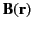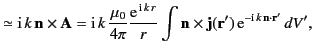(1224)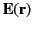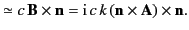(1225)

These are clearly radiation fields, because they are mutually orthogonal, transverse to the radius vector,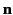, and satisfy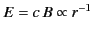. (See Section 1.8.) The three constraints (1221), (1223), and (1225), can be summed up in the single inequality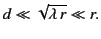(1226)

The current density associated with a linear, sinusoidal, centre-fed antenna, aligned along the-axis, is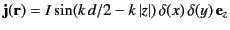(1227)

for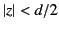, with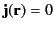for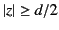. In this case, Equation (1224) yields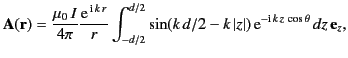(1228)

where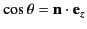. The result of this straightforward integration is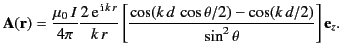(1229)

According to Equations (1226) and (1227), the electric component of the emitted radiation lies in the plane containing the antenna and the radius vector connecting the antenna to the observation point. The time-averaged power radiated by the antenna per unit solid angle is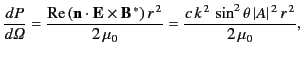(1230)

or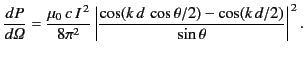(1231)

The angular distribution of power depends on the value of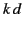. In the long wavelength limit,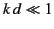, the distribution reduces to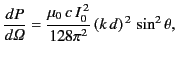(1232)

where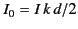is the peak current in the antenna. It is easily shown, from Equation (1229), that the associated current distribution in the antenna is linear: that is,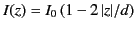(1233)

for. This type of antenna corresponds to a short (compared to the wavelength) oscillating electric dipole, and is generally known as a Hertzian dipole. The total power radiated is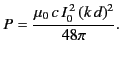(1234)

In order to maintain the radiation, power must be supplied continuously to the dipole from some generator. By analogy with the heating power produced in a resistor,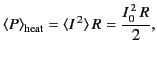(1235)

we can define the factor which multiplies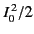in Equation (1236) as the radiation resistance of the dipole antenna. Hence,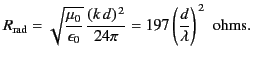(1236)

Because we have assumed that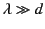, this radiation resistance is necessarily small. Typically, in a Herztian dipole, the radiated power is swamped by ohmic losses that appear as heat. Thus, a short'' antenna is a very inefficient radiator. Practical antennas have dimensions that are comparable with the wavelength of the emitted radiation.

Probably the two most common practical antennas are the half-wave antenna (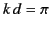) and the full-wave antenna (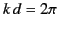). In the former case, Equation (1233) reduces to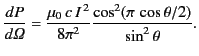(1237)

In the latter case, Equation (1233) yields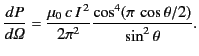(1238)

The half-wave antenna radiation pattern is very similar to the characteristic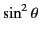pattern of a Hertzian dipole. However, the full-wave antenna radiation pattern is considerably sharper (i.e., it is more concentrated in the transverse directions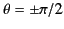).

The total power radiated by a half-wave antenna is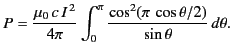(1239)

The integral can be evaluated numerically to give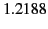. Thus,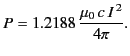(1240)

Note, from Equation (1229), that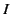is equivalent to the peak current flowing in the antenna. Thus, the radiation resistance of a half-wave antenna is given by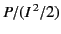, or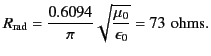(1241)

This resistance is substantially larger than that of a Hertzian dipole [see Equation (1238)]. In other words, a half-wave antenna is a far more efficient emitter of electromagnetic radiation than a Hertzian dipole. According to standard transmission line theory, if a transmission line is terminated by a resistor whose resistance matches the characteristic impedance of the line then all of the power transmitted down the line is dissipated in the resistor. On the other hand, if the resistance does not match the impedance of the line then some of the power is reflected and returned to the generator. We can think of a half-wave antenna, centre-fed by a transmission line, as a 73 ohm resistor terminating the line. The only difference is that the power absorbed from the line is radiated rather than dissipated as heat. Thus, in order to avoid problems with reflected power, the impedance of a transmission line feeding a half-wave antenna must be 73 ohms. Not surprisingly, 73 ohm impedance is one of the standard ratings for the co-axial cables used in amateur radio.Next: Antenna Directivity and Effective Up: Radiation and Scattering Previous: Introduction
Richard Fitzpatrick 2014-06-27